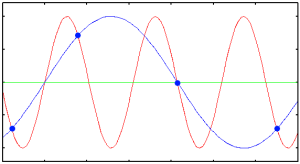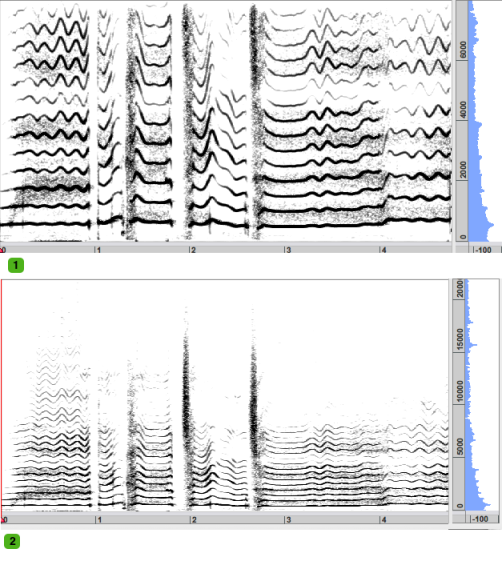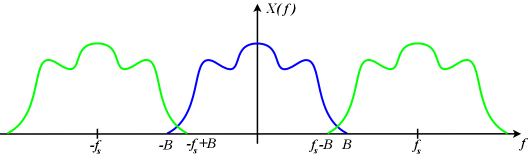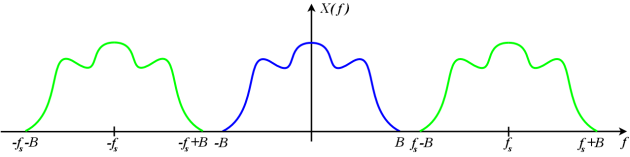IntroductionSignal Analysis > Signal Analysis Introduction > Preliminary Notions > Aliasing
page précédentepage suivante

# Aliasing

## Definition

AliasingThe blue dots are samples whose frequency is that of the sampling rate. The frequency isn't high enough to reconstruct the original signal, and produces a blue wrong signal. Aliasing  – from alias – is an effect that makes different signals indistinguishable when sampled. It also refers to the difference between a signal reconstructed from samples and the original continuous signal, when the resolution is too low. Basically, aliasing depends on the sampling rate and freqency content of the signal.
Nyquist-Shannon Theorem

The sampling rate must be equal or superior to the double of the highest frequency or the signal.

SR = Fmax * 2

A signal is bandlimited if it contains no energy above some bandlimit B. The signal is constrained in how rapidly it changes in time. The Nyquist criterion presumes that the frequency content of the signal has an upper bound, and that the signal's duration has no upper bound. We have a mathematical model that is an approximation for real samples of a real signal.1. A sound was originally sampled at 44.1 KHz. The components up to 22.05 KHz are represented. 2. The same sound resampled at a 16 KHz frequency. The components above 8 KHz are not represented.

## Aliasing and Sampling Rate

Sampling Rate and Nyquist Frequency Limit

▪ For a given highest frequency B, we get the lower bound on the sampling frequency : 2B or Nyquist rate. For instance : for a signal whose maximum frequency is 16 KHz, we need a 32 KHz sampling rate.

▪ For a given sampling rate, we get the upper bound for frequency components : B<fs/2, or Nyquist frequency or Fmax. For instance : for a signal whose sampling rate is 48 KHz, we can sample signals up to 24 KHz.

In practice, a signal can never be perfectly bandlimited. Even if an ideal reconstruction could be made, the reconstructed signal would not be exactly the original signal. The error that corresponds to the failure of bandlimitation is referred to as aliasing.The blue sampled signal is insufficiently bandlimited. The overlapping edges of the green images are added and creating a spectrum.

When a signal is sampled, its contents is reduced from real numbers to integer numbers. Values can be rounded to a superior or inferior value.

If a signal is sampled with a 32 KHz sampling rate, any frequency components above 16 KHz – Nyquist frequency, create an aliasing.

Any frequency component above SR/2 is indistinguishable from a lower-frequency component, called an alias, associated with one of the copies.

The Fourier transfom of the signal creates a symetrical image. The energy above the Nyquist frequency is transfered below this frequency.The blue signal is bandlimited and properly sampled. The images do not overlap.
Anti Aliasing Filters

Anti-aliasing filters allow to remove components above the Nyquist frequency prior to sampling. Low-frequency aliases are still generated, but at very low amplitude levels and can be reconstructed without significant additional distortion. In the example above, an anti-aliasing filter has been used to convert the file at a new sampling rate.

page précédentepage suivante